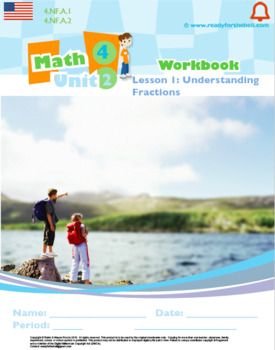# Grade 4: Math: Decimals & Fractions Worksheet BundleSubject
Resource Type
File Type

Zip

(51 MB|96 pages)
Product Rating
Standards
6 Products in this Bundle
6 products
Also included in:
1. Ready for the Bell math resources provide a comprehensive set of print, multimedia resources, and assessments with a real world learning explorations.There are several types of activities in the Student Worksheets  that can be used for formative or summative assessment. They include:-Let’s Explore (
\$93.00
\$83.70
Save \$9.30
• Bundle Description
• StandardsNEW

Ready for the Bell math resources provide a comprehensive set of print, multimedia resources, and assessments with real world learning explorations.

There are several types of activities in the Student Worksheets  that can be used for formative or summative assessment. They include:

-Let’s Explore (Exploration activities) – hands on active learning activities

-Let’s Practice – additional activities for students to practice

- Mixed Review – a series of questions that have been developed based on what students will have learned by this point as well as some questions that hit at areas that will be emerging in the future. You can use these Mixed Review questions as a formative assessment strategy to help you see the level of student understanding. You can also use some of these questions as an opportunity to extend the concept further with your students.

This Worksheet includes:

- 6 sets of Student Worksheets: 48 pages total

- 6 sets of Answer Key for Worksheets: 48 pages total

This Worksheet is part of the Math 4 lesson bundles. Click below to see these bundles.

Unit Packet: Decimals and Fractions

Common Core Alignment

CCSS.MATH.CONTENT.4.NF.A.1
Explain why a fraction a/b is equivalent to a fraction (n × a)/(n × b) by using visual fraction models, with attention to how the number and size of the parts differ even though the two fractions themselves are the same size. Use this principle to recognize and generate equivalent fractions.

CCSS.MATH.CONTENT.4.NF.A.2
Compare two fractions with different numerators and different denominators, e.g., by creating common denominators or numerators, or by comparing to a benchmark fraction such as 1/2. Recognize that comparisons are valid only when the two fractions refer to the same whole. Record the results of comparisons with symbols >, =, or <, and justify the conclusions, e.g., by using a visual fraction model.

CCSS.MATH.CONTENT.4.NF.C.5
Express a fraction with denominator 10 as an equivalent fraction with denominator 100, and use this technique to add two fractions with respective denominators 10 and 100.2 For example, express 3/10 as 30/100, and add 3/10 + 4/100 = 34/100.

CCSS.MATH.CONTENT.4.NF.C.6
Use decimal notation for fractions with denominators 10 or 100. For example, rewrite 0.62 as 62/100; describe a length as 0.62 meters; locate 0.62 on a number line diagram.

CCSS.MATH.CONTENT.4.NF.C.7
Compare two decimals to hundredths by reasoning about their size. Recognize that comparisons are valid only when the two decimals refer to the same whole. Record the results of comparisons with the symbols >, =, or <, and justify the conclusions, e.g., by using a visual model.

Compare two decimals to hundredths by reasoning about their size. Recognize that comparisons are valid only when the two decimals refer to the same whole. Record the results of comparisons with the symbols >, =, or <, and justify the conclusions, e.g., by using a visual model.
Use decimal notation for fractions with denominators 10 or 100. For example, rewrite 0.62 as 62/100; describe a length as 0.62 meters; locate 0.62 on a number line diagram.
Express a fraction with denominator 10 as an equivalent fraction with denominator 100, and use this technique to add two fractions with respective denominators 10 and 100. For example, express 3/10 as 30/100, and add 3/10 + 4/100 = 34/100.
Compare two fractions with different numerators and different denominators, e.g., by creating common denominators or numerators, or by comparing to a benchmark fraction such as 1/2. Recognize that comparisons are valid only when the two fractions refer to the same whole. Record the results of comparisons with symbols >, =, or <, and justify the conclusions, e.g., by using a visual fraction model.
Explain why a fraction 𝘢/𝘣 is equivalent to a fraction (𝘯 × 𝘢)/(𝘯 × 𝘣) by using visual fraction models, with attention to how the number and size of the parts differ even though the two fractions themselves are the same size. Use this principle to recognize and generate equivalent fractions.
Total Pages
96 pages
Included
Teaching Duration
2 months
Report this Resource to TpT
Reported resources will be reviewed by our team. Report this resource to let us know if this resource violates TpT’s content guidelines.